# GSEB Solutions Class 10 Maths Chapter 9 Some Applications of Trigonometry Ex 9.1

Gujarat Board GSEB Solutions Class 10 Maths Chapter 9 Some Applications of Trigonometry Ex 9.1 Textbook Questions and Answers.

## Gujarat Board Textbook Solutions Class 10 Maths Chapter 9 Some Applications of Trigonometry Ex 9.1

Question 1.
A circus artist is climbing a 20 m long rope, which is tightly stretched and tied from the top of a vertical pole to the ground. Find the height of the pole, if the angle made by the rope with the ground level is 300.
Solution:
In right ΔABCsin θ = $$\frac {AB}{BC}$$
⇒ sin30° = $$\frac {AB}{20}$$
⇒ $$\frac {1}{2}$$ = $$\frac {AB}{20}$$
⇒ AB = $$\frac {20}{2}$$
AB = 10m

Question 2.
A tree breaks due to a storm and the broken part bends so that the top of the tree touches the ground making an angle 30° with it. The distance between the foot of the tree to the point where the top touches the ground is 8 m. Find the height of the tree.
Solution:
Let actual height of tree be BD = AB + AD
But tree breaks and upper end D touches the ground at point CNow in ΔABC,
⇒ cos θ = $$\frac {BC}{AC}$$
⇒ cos 30° = $$\frac {8}{AC}$$
⇒ $$\frac{\sqrt{3}}{2}$$ = $$\frac {8}{AC}$$
⇒ AC = $$\frac{16}{\sqrt{3}}$$ m ………(1)Again, in ΔABC
tan 30° = $$\frac {AB}{BC}$$
⇒ $$\frac{1}{\sqrt{3}}$$ = $$\frac {AB}{8}$$
⇒ AB = $$\frac{8}{\sqrt{3}}$$ ………(2)
Height of tree BD = $$\frac{16}{\sqrt{3}}$$ + $$\frac{8}{\sqrt{3}}$$
= $$\frac{24}{\sqrt{3}}$$ = $$\frac{24}{\sqrt{3}}$$ x $$\frac{\sqrt{3}}{\sqrt{3}}$$
= $$\frac{24 \sqrt{3}}{3}$$ = $$8 \sqrt{3}$$ m

Question 3.
A contractor plans to install two slides for the children to play in a park below the age of 5 years, she prefers to have a slide whose top is at a height of 1.5 m, and is inclined at an angle of 300 to the ground, whereas for elder children, she wants to have a steep slide at a height of 3 m, and inclined at an angle of 60° to the ground. What should be the length of the slide in each case?
Solution:For children below the age 5 years
In right ΔABC
sin θ = $$\frac {AB}{AC}$$
⇒ sin 30° = $$\frac {1.5}{AC}$$
$$\frac {1}{2}$$ = $$\frac {1.5}{AC}$$
⇒ AC = 1.5 x 2 = 3 m
For elder children
In right ΔDEF
sin 60° = $$\frac {DE}{DF}$$
⇒ $$\frac{\sqrt{3}}{2}$$ = $$\frac {3}{DF}$$
DF = $$\frac{6}{\sqrt{3}}$$
DF = $$\frac{6 \sqrt{3}}{\sqrt{3} \times \sqrt{3}}$$ = $$\frac{6 \sqrt{3}}{3}$$
DF = $$2 \sqrt{3}$$ = 2 x 1.732 = 3. 464m
⇒ DF = 3.46 m (approx.)Question 4.
The angle of elevation of the top of a tower from a point on the ground which is 30 m away from the foot of the tower is 30°. Find the height of the tower.
Solution: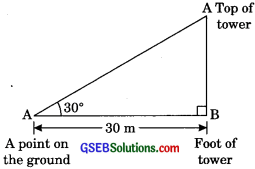In right ΔABC
tan θ = $$\frac {AB}{BC}$$
tan 30° = $$\frac {AB}{30}$$
⇒ $$\frac{1}{\sqrt{3}}$$ = $$\frac {AB}{30}$$
⇒ AB = $$\frac{30}{\sqrt{3}}$$
⇒ AB = $$\frac{30}{\sqrt{3}}$$ x $$\frac{3}{\sqrt{3}}$$ = $$\frac{30 \sqrt{3}}{3}$$
⇒ AB = $$10 \sqrt{3}$$ = 10 x 1.732
⇒ AB = 17.32 m
Hence, height of tower is 10$$\sqrt{3}$$m or 17.32 m.

Question 5.
A kite is flying at a height of 60 m above the ground. The string attached to the kite is temporarily tied to a point on the ground. The inclination of the string with the ground is 60°. Find the length of the string, assuming that there is no stack in the string.
Solution: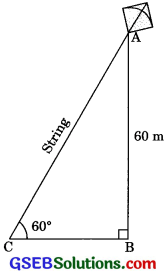In right ΔABC
sin θ = $$\frac {AB}{BC}$$
⇒ sin60° = $$\frac {60}{AC}$$
⇒ $$\frac{\sqrt{3}}{2}$$ = $$\frac {60}{AC}$$
⇒ AC = $$\frac{120}{\sqrt{3}}$$
⇒ AC = $$\frac{120}{\sqrt{3}}$$ x $$\frac{\sqrt{3}}{\sqrt{3}}$$
AC = $$\frac{120}{\sqrt{3}}$$
⇒ AC = $$40 \sqrt{3}$$
Hence, length of the string is 40J m.Question 6.
A 1.5 m tall boy is standing at some distance from a 30 m tall building. The angle of elevation from his eyes to the top of the building increases from 30° to 60° as he walks towards the building. Find the distance he walked towards the building.
Solution:
AF be the height of the building.
And height of boy = 1.5 m
AB = AF – BF
AB = 30 – 1.5 = 28.5 m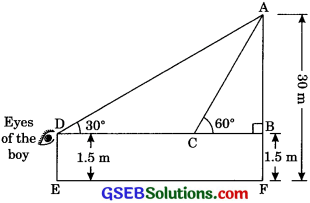In right ΔABC
tan θ = $$\frac {AB}{BC}$$
⇒ tan 60° = $$\frac{28.5}{\mathrm{BC}}$$
⇒ $$\sqrt{3}$$ = $$\frac{28.5}{\mathrm{BC}}$$
BC = $$\frac{28.5}{\mathrm{BC}}$$ m ……….(1)

Now in right ΔABD
tan θ = $$\frac {AB}{BC}$$
⇒ tan 30° = $$\frac {AB}{BC}$$
⇒ $$\frac{1}{\sqrt{3}}$$ = $$\frac{28.5}{\mathrm{BD}}$$
⇒ BD = 28.5 x $$\sqrt{3}$$
⇒ BC + CD = 28.5 x $$\sqrt{3}$$
⇒ $$\frac{28.5}{\sqrt{3}}$$ + CD = 28.5 x $$\sqrt{3}$$
⇒ CD = 28.5$$\sqrt{3}$$ – $$\frac{28.5}{\sqrt{3}}$$
⇒ CD = $$\frac{28.5 \times \sqrt{3} \times \sqrt{3}-28.5}{\sqrt{3}}$$
= $$\frac{28.5 \times 3-28.5}{\sqrt{3}}$$ = $$\frac{57}{\sqrt{3}}$$
= $$\frac{57}{\sqrt{3}}$$ x $$\frac{\sqrt{3}}{\sqrt{3}}$$ = $$\frac{57 \sqrt{3}}{3}$$ = 19$$\sqrt{3}$$ m
Hence, the distance walked towards the building is 19$$\sqrt{3}$$ m.

Question 7.
From a point on the ground, the angles of elevation of the bottom and top of a transmission tower fixed at the top of a 20 m high building are 45° and 60° respectively, find the height of the tower.
Solution: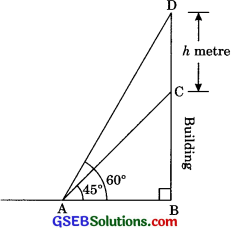In right ΔABC
tan 45° = $$\frac {BC}{AB}$$
⇒ 1 = $$\frac {20}{AB}$$
⇒ AB = 20 m ………(1)

In right ΔABD,
tan θ = $$\frac {BD}{AB}$$
⇒ tan 60° = $$\frac{20+h}{20}$$
⇒ $$\sqrt{3}$$ = $$\frac{20+h}{20}$$
⇒ 20 + h = 20$$\sqrt{3}$$
⇒ h = 20$$\sqrt{3}$$ – 20
=20 x (1.732 – 1)
= 10 x 1.464 = 14.64 m
Height of tower = 14.64 metreQuestion 8.
A statue 1.6 m tall stands on the top of a pedestal. From a point on the ground. The angle of elevation of the top of the statue is 60° and from the same point the angle of elevation of the top of the pedestal is 45° Find the height of the pedestal.
Solution:
Let the height of pedestal be h metre and AB be the statue.
In right ΔBCD
tan θ = $$\frac {BC}{DC}$$
⇒ h = x ………(1)Now in right ΔACD
tan θ = $$\frac {AC}{DC}$$
⇒ tan 60° = $$\frac{1.6 + h}{x}$$
⇒ $$\sqrt{3}$$ = $$\frac{1.6 + h}{x}$$ ………..(2)
From eqn. (1) and (2)
⇒ $$\sqrt{3}$$ = $$\frac{1.6 + h}{x}$$
⇒ $$\sqrt{3}$$h = 1.6 + h
⇒ $$\sqrt{3}$$h – h = 1.6
⇒ ($$\sqrt{3}$$)h = 1.6
h = $$\frac{1.6}{\sqrt{3} – 1}$$
h = $$\frac{1.6}{\sqrt{3} – 1}$$ x $$\frac{(\sqrt{3} + 1)}{(\sqrt{3} + 1)}$$
h = $$\frac{1.6(\sqrt{3} – 1)}{2}$$
h = 0.8($$\sqrt{3} + 1$$)
Hence, the height of the pedestal is 0.8 ($$\sqrt{3} + 1$$) metre.Question 9.
The angle of elevation of the top of a building from the foot of the tower is 30° and the angle of elevation of the top of the tower from the foot of the building is 60°. If the tower is 50 m high, find the height of the building. (CBSE 2009)
Solution:
Let height of the building be h metre.
Then, AB = h metre.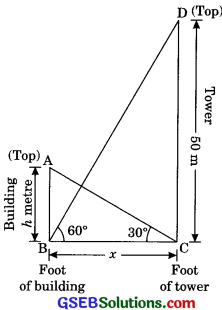In right ΔBCD
tan θ = $$\frac { CD }{ BC }$$
⇒ tan 60° = $$\frac {50}{x}$$
⇒ $$\sqrt{3}$$ = $$\frac {50}{x}$$
⇒ x = $$\frac{50}{\sqrt{3}}$$  ………..(1)

Now in right ΔACD
tan θ = $$\frac {AB}{BC}$$
⇒ tan 30° = $$\frac {h}{x}$$
⇒ $$\frac{1}{\sqrt{3}}$$ = $$\frac {h}{x}$$
⇒ x = $$\sqrt{3} h$$ ……..(2)
From (1) and (2)
⇒ $$\sqrt{3} h$$ = $$\sqrt{3} h$$
⇒ h = $$\frac{50}{\sqrt{3} \times \sqrt{3}}$$
⇒ h = $$\frac{50}{3}$$ m = 16$$\frac{2}{3}$$m
Hence, the height of the building is 16 $$\frac{2}{3}$$ metre.Question 10.
Two poles of equal heights are standing opposite each other on either side of the road, which is 80 m wide. From a point between them on the road, the angles of elevation of the top of the poles are 600 and 30°, respectively. Find the height of the poles and the distances of the point from the poles.
Solution:
Let AB and CD be the two poles.
In right ΔABC
tan θ = $$\frac {AB}{BC}$$
tan 30° = $$\frac{h}{80-x}$$
$$\frac{1}{\sqrt{3}}$$ = $$\frac{h}{80-x}$$
= 80 – x = $$\sqrt{3} h$$ ……….(1)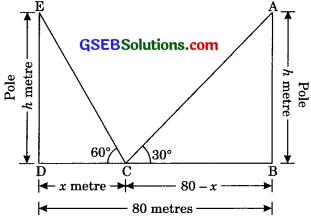Now in right ΔCDE
tan θ = $$\frac {DE}{CD}$$
⇒ tan 60° = $$\frac{h}{x}$$
⇒ $$\sqrt{3}$$ = $$\frac{h}{x}$$
h = $$\sqrt{3} x$$
From eqn. (1) and (2)
⇒ 80 – x = $$\sqrt{3} h$$
⇒ 80 – x = 3x
⇒ 4x = 80
⇒ x = $$\frac {80}{4}$$ = 20 m
Putting eqn. (2)
h = $$\sqrt{3} x$$ = $$\sqrt{3}$$ x 20 = 20$$\sqrt{3}$$ m
Height of poles be 20 m and the distance of the point from the pole is 20 m and 80 – x = 80 – 20 = 60 m.Question 11.
A TV tower stands vertically on the banks of a canal. From a point on the other bank directly opposite the tower, the angle of elevation of the top of the tower is 60°. From another point 20 be away from this point on the line joining this point to the foot of the tower, the angle of elevation of the top of the tower is 30°. Find the height of the tower and the width of the canal.
Solution:
In right ΔABC
tan θ = $$\frac{AB}{BC}$$
⇒ tan 60° = $$\frac{h}{x}$$
⇒ $$\sqrt{3}$$ = $$\frac{h}{x}$$
⇒ h = $$\sqrt{3}$$ x ………..(1)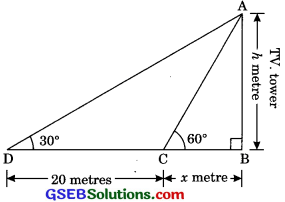Now, in right ΔABD
tan θ = $$\frac{AB}{BD}$$ (BD = x + 20)
tan 30° = $$\frac{h}{x + 20}$$
⇒ $$\frac{1}{\sqrt{3}}$$ = $$\frac{h}{x + 20}$$
⇒ x + 20 = $$\sqrt{3}$$h ……..(2)
From eqn. (1) and (2)
⇒ x + 20 = $$\sqrt{3}$$ x $$\sqrt{3}$$x
⇒ x + 20 = 3x
⇒ 2x = 20
⇒ x = 10 m
Putting value of x in eqn. (1),
h = $$\sqrt{3}$$x = $$\sqrt{3}$$ x 10
h = 10$$\sqrt{3}$$ m
Hence, the height of the tower is 10$$\sqrt{3}$$ m and the width of the canal is 10 m.Question 12.
From the top of 7 m high building, the angle of elevation of the top of a cable tower is 60° and the angle of depression of its foot is 45° Determine the height of the tower. (CBSE 2017)
Solution:
Let AC be the cable tower and DE be the building.
tan θ = $$\frac{DE}{AE}$$
⇒ tan 45° = $$\frac{7}{x}$$ ……….(1)
⇒ 1 = $$\frac{7}{x}$$
x = 7 m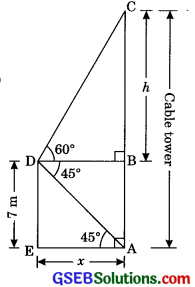Now in ΔABC
tan θ = $$\frac{BC}{BD}$$
tan 60° = $$\frac{BC}{BD}$$
$$\sqrt{3}$$ = $$\frac{h}{x}$$ (BD = EA = x)
⇒ h = $$\sqrt{3}$$x
⇒ h = $$\sqrt{3}$$ x 7 [From (1)]
⇒ h = 7$$\sqrt{3}$$ m
Height of cable tower AC
= AB + BC = 7 + 7 $$\sqrt{3}$$ = 7($$\sqrt{3}$$ + 1)
Hence height of the tower is 7($$\sqrt{3}$$ + 1) m.Question 13.
As observed from the top of a 75 m high light house from the sea level, the angles of depression of two ships are 300 and 45°. if one ship is exactly behind the other on the saine side of the light house, find the distance between the two ships.
Solution:
In right ΔABC
tan 45°= $$\frac{AB}{BC}$$
⇒ 1 = $$\frac{75}{BC}$$
⇒ BC = 75 mIn right ΔABD
tan 30° = $$\frac{AB}{BD}$$
⇒ $$\frac{1}{\sqrt{3}}$$ = $$\frac{75}{BD}$$ (AB = 75m)
⇒ BD = 75$$\sqrt{3}$$ m
CD = BD – BC
= 75$$\sqrt{3}$$ – 75 = 75($$\sqrt{3}$$ – 1) m
Distance between two ships is 75($$\sqrt{3}$$ – 1) m.Question 14.
A 12 m tall girl spots a balloon moving with the wind in a horizontal line at a height of 88.2 m from the ground. The angle of elevation of the balloon from the eyes of the girl at any instant is 600. After some time, the angle of elevation reduces to 300 (see figure). Find the distance travelled by the balloon during the interval.Solution:
Let AB = 88.2 – 1.2 m = 87 m be tkhe height of balloon from the horizontal level of girl’s eyesight.
In ΔABD
tan θ = $$\frac{AB}{BD}$$
⇒ tan 30° = $$\frac{88.2}{BD}$$
⇒ $$\frac{1}{\sqrt{3}}$$ = $$\frac{87}{BD}$$
⇒ BD = 87 x $$\sqrt{3}$$ = 87$$\sqrt{3}$$m ……….(1)

Now in right ΔDCE
tan θ = $$\frac{CE}{DC}$$
⇒ tan 60° = $$\frac{87}{DC}$$
⇒ $$\sqrt{3}$$ = $$\frac{87}{DC}$$ (CE = AB = 88.2 m)
⇒ DC = $$\frac{87}{\sqrt{3}}$$= $$\frac{87}{\sqrt{3}}$$ x $$\frac{\sqrt{3}}{\sqrt{3}}$$ = $$\frac{87 \sqrt{3}}{3}$$
⇒ DC = 29$$\sqrt{3}$$ ………(2)
BC = BD – DC
= 87$$\sqrt{3}$$ – 29$$\sqrt{3}$$ = 58$$\sqrt{3}$$ m
Hence, distance travelled by balloon is 58$$\sqrt{3}$$ m.Question 15.
A straight highway leads to the foot of a tower. A man standing at the top of the tower observes a car at an angle of depression of 30°, which is approaching the foot of the tower with a uniform speed. Six seconds later, the angle of depression of the car is found to be 600. Find the time taken by the car to reach the foot of the tower from this point. (CBSE 2008, 2009)
Solution:
Let AB be the height of the tower.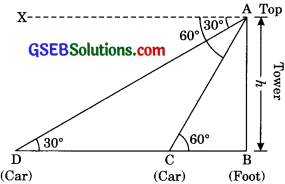In ΔABC
tan 60° = $$\frac{AB}{BC}$$
$$\sqrt{3}$$ = $$\frac{h}{BC}$$
BC = $$\frac{h}{\sqrt{3}}$$ ……….(1)

Now in ΔABD
⇒ tan θ = $$\frac{AB}{BD}$$
⇒ tan 30° = $$\frac{h}{BD}$$
⇒ $$\frac{1}{\sqrt{3}}$$ = $$\frac{h}{BD}$$
⇒ BD = $$\sqrt{3} h$$ ………..(2)
CD = BD – BC = $$\sqrt{3} h$$ – $$\frac{h}{\sqrt{3}}$$
CD = $$\frac{3 h – h}{\sqrt{3}}$$ = $$\frac{2h}{\sqrt{3}}$$
Time taken by car to travel a distance CD = $$\frac{2h}{\sqrt{3}}$$ is 6 seconds
∴ $$\frac{2h}{\sqrt{3}}$$ metre distance travelled in 6 seconds
∴ $$\frac{h}{\sqrt{3}}$$ metre distance travelled in = $$\frac{6}{2 h} \times \frac{\sqrt{3} \times h}{\sqrt{3}}=\frac{6}{2}$$ = 3 seconds
Hence, time taken by car to reach foot of tower is 3 seconds.Question 16.
The angles of elevation of the top of a tower from two points at a distance of 4 m and 9 m from the base of the tower and in the same straight line with it are complementary. Prove that the height of the tower is 6 m.
Solution:
Let h = AB be the height of tower
In right ΔABD
tan θ = $$\frac{AB}{AD}$$
tan θ = $$\frac{h}{9}$$ ………(1)Now, in ΔABC
tan (90° – θ) = $$\frac{AB}{AC}$$ ………(1)
⇒ cot θ = $$\frac{h}{4}$$ ………….(2)
From eqn. (1) and (2)
tan θ x cot θ = $$\frac{h}{4}$$ x $$\frac{h}{4}$$
tan θ x $$\frac{1}{\tan \theta}$$ = $$\frac{h^{2}}{36}$$
⇒ 1 = $$\frac{h^{2}}{36}$$
⇒ h2 = 36
⇒ h = 6
Hence, height of tower is 6 m.